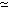SEARCH HOMEMath Central Quandaries & QueriesQuestion from Michael, a parent: My son has this question as part of his key stage 3 maths homework- The nth term of a different sequence is n - 2/n^2. We've got to find the first three terms of the sequence. I've tried to help my son with this and we can up with -1, 0, 0.1. Have we done this correctly, if not how do we work out the answerMichael,

If the n2 divides the whole expression before, that is, (n - 2)/n2,
then the first three terms are indeed
(1 - 2)/12 = -1, (2 - 2)/22 = 0,
(3 - 2)/n2 = 1/9 \simeq 0.1110.1.

But as you have written it here, I would interpret it as
n - (2/n2), whose first three terms are
1 - (2/12) = -1, 2 - (2/22) = 1.5, 3 - (2/32)2.777

ClaudeMath Central is supported by the University of Regina and The Pacific Institute for the Mathematical Sciences.